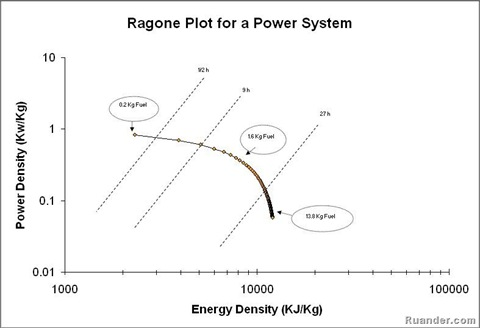## Pages

### Ragone Plot

A Ragone Plot is a tool use to evaluate the performance of a given power system. On this plot the power density is plotted against the energy density in a logarithmic scale. The power density, usually measured in kilowatts per kilogram (KW/Kg), represent how fast the power system can deliver the energy divided by how much does the power system weights. The energy density, usually measured in kilojoules per kilogram (KJ/Kg), represents the total amount of energy available divided by how much does the power system weights. Let’s use an example to better understand this plot. Consider a Brayton cycle (basically a jet engine). The total weight of this power system does not only depend on the physical set up such as the weight of the compressor, the combustor, the turbine, the fuel reservoir and so on, but it also depends on the amount and type fuel. As the fuel burns, the total weight of the power system decreases. The power capability of this power system is limited by the amount of power that the turbine can deliver. Therefore it does not matter if you have a full, half full or almost empty tank of fuel, as long as there is fuel the power that the turbine can deliver is the same. The amount of energy available in the system at any time depends on the amount of fuel. As expected, if the tank is full there is more energy in the system as compared to a half full tank. An example Ragone plot of this system is shown below:The dashed lines on the plot estimate the time that the power system can operate before it runs out of energy. For example when there are 13.8kg of fuel in the fuel tank, the system can operate for a little longer than 27 hours but its power density it’s the lowest. If the system has 0.2kg of fuel left in the tank, the system can operate for less than half an hour, but its power density it’s the best. These types of plots are used frequently in aerospace and aeronautics applications. In general, Ragone plots are applied to many different energy storage devices such as batteries, fuel cell, capacitors and so on.